# Icosagon facts for kids

Kids Encyclopedia Facts
Regular icosagon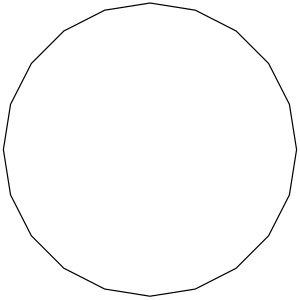A regular icosagon
Type Regular polygon
Edges and vertices 20
Schläfli symbol {20}, t{10}, tt{5}
Coxeter diagram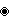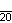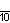Symmetry group Dihedral (D20), order 2×20
Internal angle (degrees) 162°
Dual polygon Self
Properties Convex, cyclic, equilateral, isogonal, isotoxal

An icosagon is a shape with 20 sides and 20 corners. It has interior angles of 162 and exterior angles of 198.

## Regular icosagon

The regular icosagon has Schläfli symbol {20}, and can also be constructed as a truncated decagon, t{10}, or a twice-truncated pentagon, tt{5}.

### Area

The amount of space a regular icosagon takes up is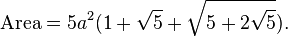$\text{Area} = {5}a^2(1+\sqrt{5}+\sqrt{5+2\sqrt{5}}).$

a is the length of one of its sides.

## Uses

The Big Wheel on the popular US game show The Price Is Right has an icosagonal cross-section.

The Globe, the outdoor theater used by William Shakespeare's acting company, was discovered to have been built on an icosagonal foundation when a partial excavation was done in 1989.

As a golygonal path, the swastika is considered to be an irregular icosagon.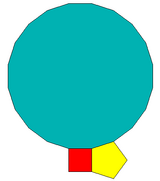A regular square, pentagon, and icosagon can completely fill a plane vertex.

## Dissection

Coxeter states that every parallel-sided 2m-gon can be divided into m(m-1)/2 rhombs. For the icosagon, m=10, and it can be divided into 45: 5 squares and 4 sets of 10 rhombs. This decomposition is based on a Petrie polygon projection of a 10-cube, with 45 of 11520 faces. The list A006245 enumerates the number of solutions as 18,410,581,880, including up to 20-fold rotations and chiral forms in reflection.

## Images for kidsIcosagon Facts for Kids. Kiddle Encyclopedia.